• 关于频谱、能量谱、功率谱对于能量信号，常用能量谱来描述。所谓的能量谱，也称为能量谱密度。是指密度的概念表示信号能量在各频率点的分布情况。也即是说，对能量谱在频域上积分就可以得到信号的能量。能量谱是...


关于频谱、能量谱、功率谱
对于能量信号，常用能量谱来描述。所谓的能量谱，也称为能量谱密度。
是指用密度的概念表示信号能量在各频率点的分布情况。也即是说，对能量谱在频域上积分就可以得到信号的能量。能量谱是信号幅度谱的模的平方，其量纲是焦/赫。
对于功率信号，常用功率谱来描述。所谓的功率谱，也称为功率谱密度。
是指用密度的概念表示信号功率在各频率点的分布情况。也就是说，对功率谱在频域上积分就可以得到信号的功率。
从理论上来说，功率谱是信号自相关函数的傅里叶变换。因为功率信号不满足傅里叶变换的条件，其频谱通常不存在，维纳-辛钦定理证明了自相关函数和傅里叶变换之间对应关系。
在工程实际中，即便是功率信号，由于持续的时间有限，可以直接对信号进行傅里叶变换，然后对得到的幅度谱的模求平方，再除以持续时间来估计信号的功率谱。
对确定性的信号，特别是非周期的确定性信号，常用能量谱来描述。而对于随机信号，由于持续期时间无限长，不满足绝对可积与能量可积的条件，因此不存在傅立叶变换，所以通常用功率谱来描述。
周期性的信号，也同样是不满足傅里叶变换的条件，常用功率谱来描述。
频谱、幅度谱、功率谱和能量谱 - 木心的木偶 - 博客园​www.cnblogs.com

关于功率谱
功率谱可以从两方面来定义：
一个是自相关函数的傅立叶变换（维纳辛钦定理）；
另一个是时域信号傅氏变换模平方然后除以时间长度（来自能量谱密度）。 根据parseval定理，信号傅氏变换模平方被定义为能量谱，能量谱密度在时间上平均就得到了功率谱。
理解：
（1）信号通常分为两类：能量信号和功率信号；
（2）一般来讲，能量信号其傅氏变换收敛（即存在），而功率信号傅氏变换通常不收敛；
（3）信号是信息的搭载工具，而信息与随机性紧密相关，所以实际信号多为随机信号，这类信号的特点是状态随机性随时间无限延伸，其样本能量无限。换句话说，随机信号（样本）大多属于功率信号而非能量信号，它并不存在傅氏变换，亦即不存在频谱；
（4）若撇开搭载信息的有用与否，随机信号又称随机过程，很多噪声属于特殊的随机过程，它们的某些统计特性具有平稳性，其均值和自相关函数具有平稳性。对于这样的随机过程，自相关函数蜕化为一维确定函数，前人证明该确定相关函数存在傅氏变换；
（5）频谱通常既含有幅度也含有相位信息；幅度谱的平方（二次量纲）又叫能量谱（密度），它描述了信号能量的频域分布；功率信号的功率谱（密度）描述了信号功率随频率的分布特点（密度：单位频率上的功率），业已证明，平稳信号功率谱密度恰好是其自相关函数的傅氏变换。对于非平稳信号，其自相关函数的时间平均（对时间积分，随时变性消失而再次退变成一维函数）与功率谱密度仍是傅氏变换对；
（6）实际中我们获得的往往仅仅是信号的一段支撑，此时即使信号为功率信号，截断之后其傅氏变换收敛，但此变换结果严格来讲不属于任何“谱”（进一步分析可知它是样本真实频谱的平滑：卷积谱）；
（7）对于（6）中所述变换若取其幅度平方再除以持续时间，可作为平稳信号功率谱（密度）的近似，是为经典的“周期图法”；
补充：（8） 一个信号的频谱,只是这个信号从时域表示转变为频域表示,只是同一种信号的不同的表示方式而已；而功率谱是从能量的观点对信号进行的研究,其实频谱和功率谱的关系归根揭底还是信号和功率,能量等之间的关系。

信号---经典谱估计（功率谱，频谱的理解） - kiki__xiunai - 博客园​www.cnblogs.com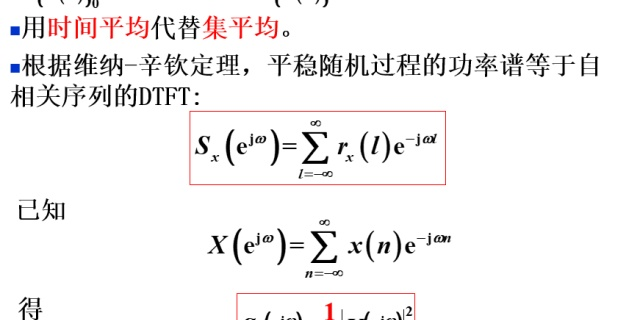关于功率谱估计
功率谱估计一般分成两大类： 经典谱估计和 现代谱估计。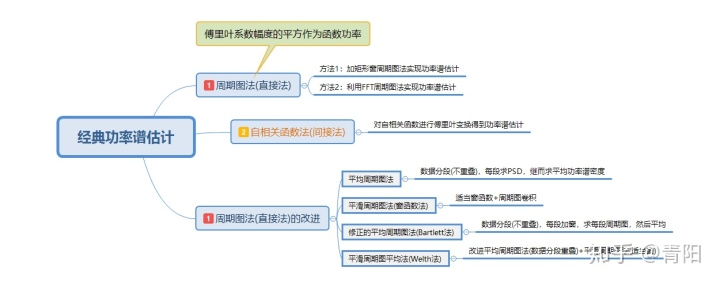经典谱估计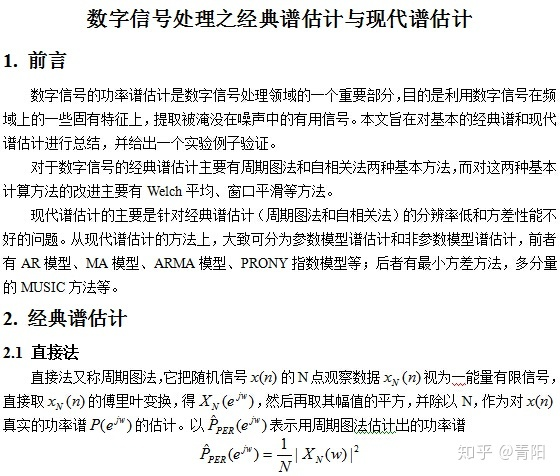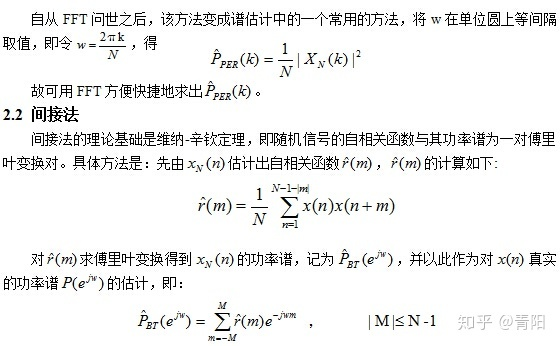自相关法
自相关法是根据维纳-辛钦定理，通过相关函数计算功率谱的。
周期图法于年提出，把点观测数据看做能量有限的信号，直接对其进行傅里叶变换，然后取其模值的平方，并除以，得到观测数据真实的功率谱的估计。

质量分析：
1.周期图法是功率谱的有偏估计。产生偏移的原因一方面是局部平均中主瓣的模糊作用，模糊的结果使谱估计的分辨率下降；另一方面是由于旁瓣的泄露。
2.频率分辨率低。原因是傅里叶变换域是无限长的，而观测数据是有限长的，相当于将信号在时域添加了矩形窗，在频域真正功率谱卷积一个抽样函数。

总结：
周期图法很简单，直接使用谱估计性能很差，因此在使用时一般使用改进的周期图法。
四种常见的改进周期图法
周期图法相当于一个矩形窗对时域信号的加窗，然后时间分辨率大致与数据长度成反比。这里提出四种常见的改进周期图法。

平均周期图法
对一个随机变量观测时，得到组独立数据，每组数据长为，对每一组求，之后将个均值加起来求平均。这样得到的均值，其方差是原来的。

质量分析：
平均周期图法仍然是有偏估计，偏移和每组数据长度有关。由于每段的长度变为，频谱分辨率更低，因此，平均周期图法以分辨率的降低换取了估计方差的减少。

窗函数法
窗函数法是将长度为的观测数据乘以同一长度的数据窗，数据加窗后，谱估计值的数学期望等于真实谱值与窗谱函数的平方卷积，因而是有偏估计。

质量分析：
选择低旁瓣的数据窗可使得杂散响应减少，但旁瓣的降低必然使得主瓣加宽，从而降低分辨率。
数据加窗后，谱估计值的方差谱估计值的平方，且不随数据长度增大而减少。

数据加窗可以降低谱估计值的旁瓣，但是降低了谱估计的分辨率。
修正的周期图平均法
又称法，首先把长度为N的数据分成段，每段数据长为，则。然后把窗函数加到每段数据上，求出每段的周期图，之后对每段周期图进行评价。

质量分析：法在计算周期图前，先对各数据段加窗，是平均周期图法的估计方差减少，但是分辨率降低。

加权交叠平均法
又称法。对法的改进。首先，分段时相邻两段可以重叠，其次，窗函数使用汉宁窗或汉明窗，通过改进，达到进一步减小方差的目的。

总结：
经典功率谱虽然实现简单，计算速度快，但是具有方差性能较差、分辨率较低、旁瓣泄露等缺点。

经典功率谱估计及其实现_我的梦-CSDN博客_功率谱估计​blog.csdn.net现代谱估计
分为参数模型估计和非参模型估计，这里主要是基于模型的谱估计。

基于模型的谱估计的几种方法：

1.求解方程

在求解方程中的系数可用递推算法简化计算，但它需要知道自相关序列。自相关序列实际上只能从随机序列的有限个观测数据估计得到。当时间序列较短时，估计误差很大，这将对AR参数的计算引入较大的误差，导致谱估计性能下降，甚至出现谱线分裂与谱峰偏移等现象。

2.法

如果利用观测到的数据直接计算模型的参数，则能克服上述方法的缺点，得到性能较好的谱估计结果。这种方法是由提出的，称为法，也叫做最大熵谱算法。递推算法的优点是不需要估计自相关函数，它直接从已知序列求得反射系数，然后利用递推算法由反射系数来求得参数。


展开全文• 周期图法是直接将信号的采样数据x(n)进行Fourier变换求取功率谱密度估计的方法。假定有限长随机信号序列为x(n)。它的Fourier变换和功率谱密度估计存在下面的关系： 式中，N为随机信号序列x(n)的长度。在离散的频率点...
1. 基本方法 周期图法是直接将信号的采样数据x(n)进行Fourier变换求取功率谱密度估计的方法。假定有限长随机信号序列为x(n)。它的Fourier变换和功率谱密度估计存在下面的关系： 式中，N为随机信号序列x(n)的长度。在离散的频率点f=kΔf,有： 其中，FFT[x(n)]为对序列x(n)的Fourier变换，由于FFT[x(n)]的周期为N，求得的功率谱估计以N为周期，因此这种方法称为周期图法。下面用例子说明如何采用这种方法进行功率谱 用有限长样本序列的Fourier变换来表示随机序列的功率谱，只是一种估计或近似，不可避免存在误差。为了减少误差，使功率谱估计更加平滑，可采用分段平均周期图法（Bartlett法）、加窗平均周期图法（Welch法）等方法加以改进。 2. 分段平均周期图法（Bartlett法） 将信号序列x(n)，n=0,1,…,N-1，分成互不重叠的P个小段，每小段由m个采样值，则P*m=N。对每个小段信号序列进行功率谱估计，然后再取平均作为整个序列x(n)的功率谱估计。 平均周期图法还可以对信号x(n)进行重叠分段，如按2:1重叠分段，即前一段信号和后一段信号有一半是重叠的。对每一小段信号序列进行功率谱估计，然后再取平均值作为整个序列x(n)的功率谱估计。这两种方法都称为平均周期图法，一般后者比前者好。程序运行结果为图9-5，上图采用不重叠分段法的功率谱估计，下图为2:1重叠分段的功率谱估计，可见后者估计曲线较为平滑。与上例比较，平均周期图法功率谱估计具有明显效果（涨落曲线靠近0dB）。 3.加窗平均周期图法 加窗平均周期图法是对分段平均周期图法的改进。在信号序列x(n)分段后，用非矩形窗口对每一小段信号序列进行预处理，再采用前述分段平均周期图法进行整个信号序列x(n)的功率谱估计。由窗函数的基本知识（第7章）可知，采用合适的非矩形窗口对信号进行处理可减小“频谱泄露”，同时可增加频峰的宽度，从而提高频谱分辨率。 其中上图采用无重叠数据分段的加窗平均周期图法进行功率谱估计，而下图采用重叠数据分段的加窗平均周期图法进行功率谱估计，显然后者是更佳的，信号谱峰加宽，而噪声谱均在0dB附近，更为平坦（注意采用无重叠数据分段噪声的最大的下降分贝数大于5dB，而重叠数据分段周期图法噪声的最大下降分贝数小于5dB）。 4. Welch法估计及其MATLAB函数 Welch功率谱密度就是用改进的平均周期图法来求取随机信号的功率谱密度估计的。Welch法采用信号重叠分段、加窗函数和FFT算法等计算一个信号序列的自功率谱估计(PSD如上例中的下半部分的求法)和两个信号序列的互功率谱估计（CSD）。 MATLAB信号处理工具箱函数提供了专门的函数PSD和CSD自动实现Welch法估计，而不需要自己编程。 （1） 函数psd利用Welch法估计一个信号自功率谱密度，函数调用格式为： [Pxx[,f]]=psd(x[,Nfft,Fs,window,Noverlap,’dflag’]) 式中，x为信号序列；Nfft为采用的FFT长度。这一值决定了功率谱估计速度，当Nfft采用2的幂时，程序采用快速算法；Fs为采样频率；Window定义窗函数和x分段序列的长度。窗函数长度必须小于或等于Nfft，否则会给出错误信息；Noverlap为分段序列重叠的采样点数（长度），它应小于Nfft;dflag为去除信号趋势分量的选择项：’linear’,去除线性趋势分量，’mean’去除均值分量，’none’不做去除趋势处理。Pxx为信号x的自功率谱密度估计。f为返回的频率向量，它和Pxx对应，并且有相同长度。 在psd函数调用格式中，缺省值为：Nfft=min(256,length(x)),Fs=2Hz, window=hanning(Nfft),noverlap=0. 若x是实序列，函数psd仅计算频率为正的功率 注意程序前半部分中频率向量f的创建方法。它与函数psd的输出Pxx长度的关系如下：若x为实序列，当Nfft为奇数时，f=(0:(Nfft+1)/2-1)/Nfft;当Nfft为偶数时，f=(0:Nfft/2)/Nfft。 函数还有一种缺省返回值的调用格式，用于直接绘制信号序列x的功率谱估计曲线。 函数还可以计算带有置信区间的功率谱估计，调用格式为： [Pxx,Pxxc,f]=psd(x,Nfft,Fs,window,Noverlap,p) 式中，p为置信区间，0<=p<=1。 由此可知，滤波器输入白噪声序列的输出信号的功率谱或自相关可以确定滤波器的频率特性。 （2）函数csd利用welch法估计两个信号的互功率谱密度，函数调用格式为： [Pxy[,f]]=csd(x,y,Nfft,Fs,window,Noverlap,’dflag’) [Pxy,Pxyc[,f]]=csd(x,y,Nfft,Fs,window,Noverlap,p) 这里，x,y为两个信号序列；Pxy为x,y的互功率谱估计；其他参数的意义同自功率谱函数psd。 可以看到，两个白噪声信号的互功率谱（上图）杂乱无章，看不出周期成分，大部分功率谱在-5dB以下。然而白噪声与带有噪声的周期信号的功率谱在其周期（频率为1000Hz）处有一峰值，清楚地表明了周期信号的周期或频率。因此，利用未知信号与白噪声信号的互功率谱也可以检测未知信号中所含有的频率成分。 5 多 窗 口 法 多窗口法(Multitaper method,简称MTM法)利用多个正交窗口（Tapers）获得各自独立的近似功率谱估计，然后综合这些估计得到一个序列的功率谱估计。相对于普通的周期图法，这种功率谱估计具有更大的自由度，并在估计精度和估计波动方面均有较好的效果。普通的功率谱估计只利用单一窗口，因此在序列始端和末端均会丢失相关信息，而且无法找回。而MTM法估计增加窗口使得丢失的信息尽量减少。 MTM法简单地采用一个参数：时间带宽积(Time-bandwidth product)NW，这个参数用以定义计算功率谱所用窗的数目，为2*NW-1。NW越大，功率谱计算次数越多，时间域分辨率越高，而频率域分辨率降低，使得功率谱估计的波动减小。随着NW增大，每次估计中谱泄漏增多，总功率谱估计的偏差增大。对于每一个数据组，通常有一个最优的NW使得在估计偏差和估计波动两方面求得折中，这需要在程序中反复调试来获得。 MATLAB信号处理工具箱中函数PMTM就是采用MTM法估计功率谱密度。函数调用格式为： [Pxx[,f]]=pmtm(x[,nw,Nfft,Fs]) 式中，x为信号序列；nw为时间带宽积，缺省值为4。通常可取2，5/2，3，7/2；Nfft为FFT长度；Fs为采样频率。 上面的函数还可以通过无返回值而绘出置信区间，如pmtm(x,nw,Nfft,Fs,’option’,p)绘制带置信区间的功率谱密度估计曲线，0<=p<=1。 6 最 大 熵 法（Maxmum entropy method， MEM法） 如上所述，周期图法功率谱估计需要对信号序列“截断”或加窗处理，其结果是使估计的功率谱密度为信号序列真实谱和窗谱的卷积，导致误差的产生。 最大熵功率谱估计的目的是最大限度地保留截断后丢失的“窗口”以外信号的信息，使估计谱的熵最大。主要方法是以已知的自相关序列rxx(0),rxx(1),…,rxx(p)为基础，外推自相关序列rxx(p+1),rxx(p+2),…，保证信息熵最大。 最大熵功率谱估计法假定随机过程是平稳高斯过程，可以证明，随机信号的最大熵谱与AR自回归（全极点滤波器）模型谱是等价的。 MATLAB信号处理工具箱提供最大熵功率谱估计函数pmem，其调用格式为： [Pxx,f,a]=pmem(x,p,Nfft,Fs,’xcorr’) 式中，x为输入信号序列或输入相关矩阵；p为全极点滤波器阶次；a为全极点滤波器模型系数向量；’xcorr’是把x认为是相关矩阵。 比较最大熵功率谱估计(MEM)和改进的平均周期图功率谱估计，可见，MEM法估计的功率谱曲线较光滑。在这一方法中，MEM法选定全极点滤波器的阶数取得越大，能够获得的窗口外的信息越多，但计算量也越大，需要根据情况折中考虑。 7 多信号分类法 MATLAB信号处理工具箱还提供另一种功率谱估计函数pmusic。该函数执行多信号分类法（multiple signal classification， Music法)。将数据自相关矩阵看成由信号自相关矩阵和噪声自相关矩阵两部分组成，即数据自相关矩阵R包含有两个子空间信息：信号子空间和噪声子空间。这样，矩阵特征值向量（Eigen vector）也可分为两个子空间：信号子空间和噪声子空间。为了求得功率谱估计，函数pmusic计算信号子空间和噪声子空间的特征值向量函数，使得在周期信号频率处函数值最大，功率谱估计出现峰值，而在其他频率处函数值最小。其调用格式为： [Pxx,f,a]=pmusic(x,p[[,thresh],Nfft,Fs,window,Noverlap]) 式中,x为输入信号的向量或矩阵；p为信号子空间维数；thresh为阈值，其他参数的意义与函数psd相同。 功率谱密度相关方法的MATLAB实现 %% %分段平均周期图法（Bartlett法） %运用信号不重叠分段估计功率谱 Nsec=256;n=0:sigLength-1;t=n/Fs; %数据点数，分段间隔，时间序列 pxx1=abs(fft(y(1:256),Nsec).^2)/Nsec; %第一段功率谱 pxx2=abs(fft(y(257:512),Nsec).^2)/Nsec; %第二段功率谱 pxx3=abs(fft(y(515:768),Nsec).^2)/Nsec; %第三段功率谱 pxx4=abs(fft(y(769:1024),Nsec).^2)/Nsec; %第四段功率谱 Pxx=10*log10((pxx1+pxx2+pxx3+pxx4)/4); %平均得到整个序列功率谱 f=(0:length(Pxx)-1)*Fs/length(Pxx); %给出功率谱对应的频率 %%plot(f(1:Nsec/2),Pxx(1:Nsec/2)); %绘制功率谱曲线 figure,plot(f(1:Nsec),Pxx(1:Nsec)); %绘制功率谱曲线 xlabel('频率/Hz');ylabel('功率谱 /dB'); title('平均周期图(无重叠) N=4*256'); grid on %% %运用信号重叠分段估计功率谱 pxx1=abs(fft(y(1:256),Nsec).^2)/Nsec; %第一段功率谱 pxx2=abs(fft(y(129:384),Nsec).^2)/Nsec; %第二段功率谱 pxx3=abs(fft(y(257:512),Nsec).^2)/Nsec; %第三段功率谱 pxx4=abs(fft(y(385:640),Nsec).^2)/Nsec; %第四段功率谱 pxx5=abs(fft(y(513:768),Nsec).^2)/Nsec; %第五段功率谱 pxx6=abs(fft(y(641:896),Nsec).^2)/Nsec; %第六段功率谱 pxx7=abs(fft(y(769:1024),Nsec).^2)/Nsec; %第七段功率谱 Pxx=10*log10((pxx1+pxx2+pxx3+pxx4+pxx5+pxx6+pxx7)/7); %功率谱平均并转化为dB f=(0:length(Pxx)-1)*Fs/length(Pxx); %频率序列 figure,plot(f(1:Nsec/2),Pxx(1:Nsec/2)); %绘制功率谱曲线 xlabel('频率/Hz'); ylabel('功率谱/dB'); title('平均周期图(重叠一半) N=1024'); grid on %% Nsec=256;n=0:sigLength-1;t=n/Fs; %数据长度，分段数据长度、时间序列 w=hanning(256); %采用的窗口数据 %采用不重叠加窗方法的功率谱估计 pxx1=abs(fft(w.*y(1:256),Nsec).^2)/norm(w)^2; %第一段加窗振幅谱平方 pxx2=abs(fft(w.*y(257:512),Nsec).^2)/norm(w)^2; %第二段加窗振幅谱平方 pxx3=abs(fft(w.*y(513:768),Nsec).^2)/norm(w)^2; %第三段加窗振幅谱平方 pxx4=abs(fft(w.*y(769:1024),Nsec).^2)/norm(w)^2; %第四段加窗振幅谱平方 Pxx=10*log10((pxx1+pxx2+pxx3+pxx4)/4); %求得平均功率谱，转换为dB f=(0:length(Pxx)-1)*Fs/length(Pxx); %求得频率序列 figure subplot(2,1,1),plot(f(1:Nsec/2),Pxx(1:Nsec/2)); %绘制功率谱曲线 xlabel('频率/Hz');ylabel('功率谱/dB'); title('加窗平均周期图(无重叠) N=4*256'); grid on %采用重叠加窗方法的功率谱估计 pxx1=abs(fft(w.*y(1:256),Nsec).^2)/norm(w)^2; %第一段加窗振幅谱平方 pxx2=abs(fft(w.*y(129:384),Nsec).^2)/norm(w)^2; %第二段加窗振幅谱平方 pxx3=abs(fft(w.*y(257:512),Nsec).^2)/norm(w)^2; %第三段加窗振幅谱平方 pxx4=abs(fft(w.*y(385:640),Nsec).^2)/norm(w)^2; %第四段加窗振幅谱平方 pxx5=abs(fft(w.*y(513:768),Nsec).^2)/norm(w)^2; %第五段加窗振幅谱平方 pxx6=abs(fft(w.*y(641:896),Nsec).^2)/norm(w)^2; %第六段加窗振幅谱平方 pxx7=abs(fft(w.*y(769:1024),Nsec).^2)/norm(w)^2; %第七段加窗振幅谱平方 Pxx=10*log10((pxx1+pxx2+pxx3+pxx4+pxx5+pxx6+pxx7)/7);%平均功率谱转换为dB f=(0:length(Pxx)-1)*Fs/length(Pxx); %频率序列 subplot(2,1,2),plot(f(1:Nsec/2),Pxx(1:Nsec/2)); %绘制功率谱曲线 xlabel('频率/Hz');ylabel('功率谱/dB'); title('加窗平均周期图(重叠一半)N=1024'); grid on %% %4分段平均周期图法（hanning窗） Nsec=256; n=0:sigLength-1; t=n/Fs; w=hanning(256); Pxx1=abs(fft(w.*y(1:256),Nsec).^2)/Nsec; Pxx2=abs(fft(w.*y(257:512),Nsec).^2)/Nsec; Pxx3=abs(fft(w.*y(513:768),Nsec).^2)/Nsec; Pxx4=abs(fft(w.*y(769:1024),Nsec).^2)/Nsec; Pxx=10*log10((Pxx1+Pxx2+Pxx3+Pxx4)/4); f=(0:length(Pxx)-1)*Fs/length(Pxx); figure subplot(2,1,1) plot(f,Pxx); xlabel('频率/Hz');ylabel('功率谱/dB'); title('Averaged Modified Periodogram (none overlap) N=4*256'); grid %4分段（2：1重叠）平均周期图法（hanning窗） Nsec=256; n=0:sigLength-1; t=n/Fs; w=hanning(256); Pxx1=abs(fft(w.*y(1:256),Nsec).^2)/Nsec; Pxx2=abs(fft(w.*y(129:384),Nsec).^2)/Nsec; Pxx3=abs(fft(w.*y(257:512),Nsec).^2)/Nsec; Pxx4=abs(fft(w.*y(385:640),Nsec).^2)/Nsec; Pxx5=abs(fft(w.*y(513:768),Nsec).^2)/Nsec; Pxx6=abs(fft(w.*y(641:896),Nsec).^2)/Nsec; Pxx7=abs(fft(w.*y(769:1024),Nsec).^2)/Nsec; Pxx=10*log10((Pxx1+Pxx2+Pxx3+Pxx4+Pxx5+Pxx6+Pxx7)/7); f=(0:length(Pxx)-1)*Fs/length(Pxx); subplot(2,1,2);plot(f,Pxx); xlabel('频率/Hz');ylabel('Power Spectrum (dB)'); title('Averaged Modified Periodogram (half overlap) N=1024'); grid %% %PSD_WELCH方法 %采样频率 Nfft=256;n=0:sigLength-1;t=n/Fs; %数据长度、时间序列 window=hanning(256); %选用的窗口 noverlap=128; %分段序列重叠的采样点数（长度） dflag='none'; %不做趋势处理 [Pxx,Pxxc,f]=psd(y,Nfft,Fs,window,noverlap,0.95); %功率谱估计,并以0.95的置信度给出置信区间，无返回值是绘制出置信区间 figure plot(f,10*log10(Pxx)); %绘制功率谱 xlabel('频率/Hz');ylabel('功率谱/dB'); title('PSD—Welch方法'); grid on %% %最大熵法（MEM法） Nfft=256;n=0:sigLength-1;t=n/Fs; %数据长度、分段长度和时间序列 window=hanning(256); %采用窗口 [Pxx1,f]=pmem(x,20,Nfft,Fs); %采用最大熵法，采用滤波器阶数14，估计功率谱 figure,subplot(2,1,1),plot(f,10*log10(Pxx1)); %绘制功率谱 xlabel('频率/Hz');ylabel('功率谱/dB'); title('最大熵法 Order=20原始信号功率谱');grid on [Pxx1,f]=pmem(y0,20,Nfft,Fs); %采用最大熵法，采用滤波器阶数14，估计功率谱 subplot(2,1,2),plot(f,10*log10(Pxx1)); %绘制功率谱 xlabel('频率/Hz');ylabel('功率谱/dB');axis([0 4000 -20 0]) title('最大熵法 Order=20滤波后的信号功率谱');grid on %% %%功率谱密度 %PSD_WELCH方法 Nfft=512;n=0:sigLength-1;t=n/Fs; %数据长度、时间序列 window=hanning(256); %选用的窗口 noverlap=128; %分段序列重叠的采样点数（长度） dflag='none'; %不做趋势处理 [Pxx,Pxxc,f]=psd(x,Nfft,Fs,window,noverlap,0.95); %功率谱估计,并以0.95的置信度给出置信区间，无返回值是绘制出置信区间 figure;subplot(211); plot(f,10*log10(Pxx)); %绘制功率谱 xlabel('频率/Hz');ylabel('功率谱/dB'); grid on;title('PSD—Welch方法的原始信号功率谱') subplot(212) [Pxx,Pxxc,f]=psd(y0,Nfft,Fs,window,noverlap,0.95); %功率谱估计,并以0.95的置信度给出置信区间，无返回值是绘制出置信区间 plot(f,10*log10(Pxx)); %绘制功率谱 xlabel('频率/Hz');ylabel('功率谱/dB');axis([0 4000 -30 0]) grid on;title('PSD—Welch方法的滤波后的信号功率谱') %% %用多窗口法(MTM) n=0:sigLength-1;t=n/Fs; %数据长度、分段数据长度，时间序列 [Pxx1,f]=pmtm(x,2,Nfft,Fs); %用多窗口法(NW=4)估计功率谱 figure;subplot(2,1,1),plot(f,10*log10(Pxx1)); %绘制功率谱 xlabel('频率/Hz');ylabel('功率谱/dB'); title('多窗口法(MTM) nw=2原始信号功率谱');grid on [Pxx,f]=pmtm(y0,2,Nfft,Fs); %用多窗口法(NW=2)估计功率谱 subplot(2,1,2),plot(f,10*log10(Pxx)); %绘制功率谱 xlabel('频率/Hz');ylabel('功率谱/dB');axis([0 4000 -80 -20]) title('多窗口法(MTM) nw=2滤波后的信号功率谱');grid on %% %采用Welch方法估计功率谱 noverlap=128; %重叠数据 dflag='none'; Nfft=1024; figure;subplot(2,1,1) psd(x,Nfft,Fs,window,noverlap,dflag); %采用Welch方法估计功率谱 xlabel('频率/Hz');ylabel('功率谱/dB') title('Welch方法原始信号功率谱');grid on subplot(2,1,2) psd(y0,Nfft,Fs,window,noverlap,dflag); %采用Welch方法估计功率谱 xlabel('频率/Hz');ylabel('功率谱/dB'),axis([0 4000 -30 0]) title('Welch方法滤波后的信号功率谱');grid on pmusic(y0,[7,1.1],Nfft,Fs,32,16); %采用多信号分类法估计功率谱 xlabel('频率/Hz'); ylabel('功率谱/dB') title('通过MUSIC法估计的伪谱')
展开全文• 3 matlab噪声的自相关函数和功率谱密度及功率谱估计
• ## Matlab 实现经典功率谱分析和估计

万次阅读 多人点赞 2019-07-07 11:24:29
Matlab 实现经典功率谱分析和估计 文章目录Matlab 实现经典功率谱分析和估计功率谱Matlab 使用1 直接法2 间接法3 改进直接法：Bartlett法4 Welch法附上谋篇论文，分析EEG信号功率谱代码致谢 功率谱 功率谱是...
Matlab 实现经典功率谱分析和估计

文章目录
Matlab 实现经典功率谱分析和估计功率谱Matlab 使用1 直接法2 间接法3 改进直接法：Bartlett法4 Welch法
附上谋篇论文，分析EEG信号功率谱代码致谢

功率谱
功率谱是功率谱密度函数的简称，它定义为单位频带内的信号功率。它表示了信号功率随着频率的变化情况，即信号功率在频域的分布状况。功率谱表示了信号功率随着频率的变化关系 。 常用于功率信号（区别于能量信号）的表述与分析，其曲线（即功率谱曲线）一般横坐标为频率，纵坐标为功率。周期性连续信号x(t)的频谱可表示为离散的非周期序列

X

n

Xn

,它的幅度频谱的平方

│

X

n

│

2

│Xn│2

所排成的序列，就被称之为该周期信号的“功率谱”。
Matlab 使用
fft做出来是频谱，psd做出来是功率谱；功率谱丢失了频谱的相位信息；频谱不同的信号其功率谱是可能相同的；功率谱是幅度取模后平方，结果是个实数。matlab中自功率谱密度直接用psd函数就可以求，按照matlab的说法，psd能实现Welch法估计，即相当于用改进的平均周期图法来求取随机信号的功率谱密度估计。psd求出的结果应该更光滑吧。
1 直接法
直接法又称周期图法，它是把随机序列

x

(

n

)

x(n)

的

N

N

个观测数据视为一能量有限的序列，直接计算

x

(

n

)

x(n)

的离散傅立叶变换，得

X

(

k

)

X(k)

，然后再取其幅值的平方，并除以

N

N

，作为序列

x

(

n

)

x(n)

真实功率谱的估计。 Matlab 代码示例：
clear;
Fs=1000; %采样频率
n=0:1/Fs:1;
%产生含有噪声的序列
xn=cos(2*pi*40*n)+3*cos(2*pi*100*n)+randn(size(n));
window=boxcar(length(xn)); %矩形窗
nfft=1024;
[Pxx,f]=periodogram(xn,window,nfft,Fs); %直接法

subplot(1,2,1);
plot(xn);
subplot(1,2,2);
plot(f,10*log10(Pxx));

结果，左图原始信号，右图为周期图法信号。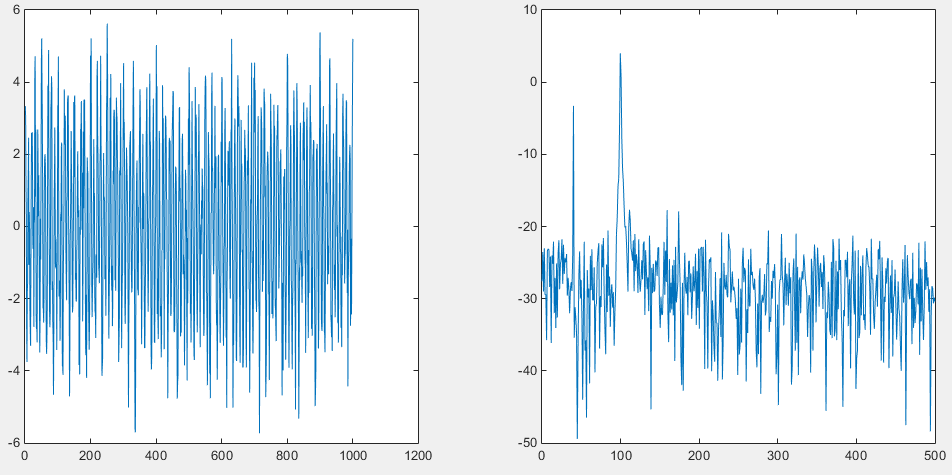2 间接法
间接法先由序列

x

(

n

)

x(n)

估计出自相关函数

R

(

n

)

R(n)

，然后对

R

(

n

)

R(n)

进行傅立叶变换，便得到

x

(

n

)

x(n)

的功率谱估计。 Matlab 代码示例：
clear;
Fs=1000; %采样频率
n=0:1/Fs:1;
%产生含有噪声的序列
xn=cos(2*pi*40*n)+3*cos(2*pi*100*n)+randn(size(n));
nfft=1024;
cxn=xcorr(xn,'unbiased'); %计算序列的自相关函数
CXk=fft(cxn,nfft);
Pxx=abs(CXk);
index=0:round(nfft/2-1);
k=index*Fs/nfft;
plot_Pxx=10*log10(Pxx(index+1));
subplot(1,2,1);
plot(xn);
subplot(1,2,2);
plot(k,plot_Pxx);

结果，右图为间接法。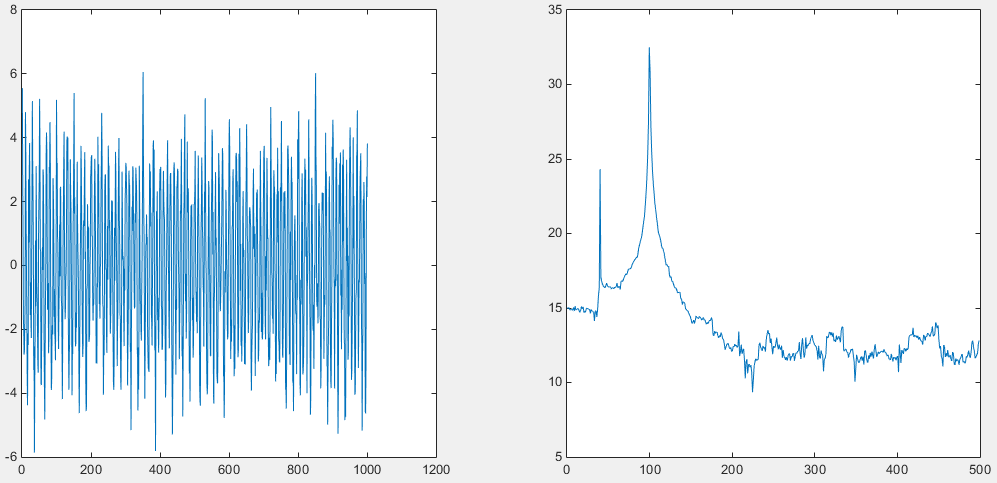3 改进直接法：Bartlett法

对于直接法的功率谱估计，当数据长度N太大时，谱曲线起伏加剧，若N太小，谱的分辨率又不好，因此需要改进。

Bartlett平均周期图的方法是将

N

N

点的有限长序列

x

(

n

)

x(n)

分段求周期图再平均。 Matlab代码示例：
clear;
Fs=1000;
n=0:1/Fs:1;
xn=cos(2*pi*40*n)+3*cos(2*pi*100*n)+randn(size(n));
nfft=1024;
window=boxcar(length(n)); %矩形窗
noverlap=0; %数据无重叠
p=0.9; %置信概率
[Pxx,Pxxc]=psd(xn,nfft,Fs,window,noverlap,p);
index=0:round(nfft/2-1);
k=index*Fs/nfft;
plot_Pxx=10*log10(Pxx(index+1));
plot_Pxxc=10*log10(Pxxc(index+1));
subplot(1,2,1);
plot(k,plot_Pxx);
subplot(1,2,2);
plot(k,[plot_Pxx plot_Pxx-plot_Pxxc plot_Pxx+plot_Pxxc]);

结果，左图为直接法，右图为Bartlett法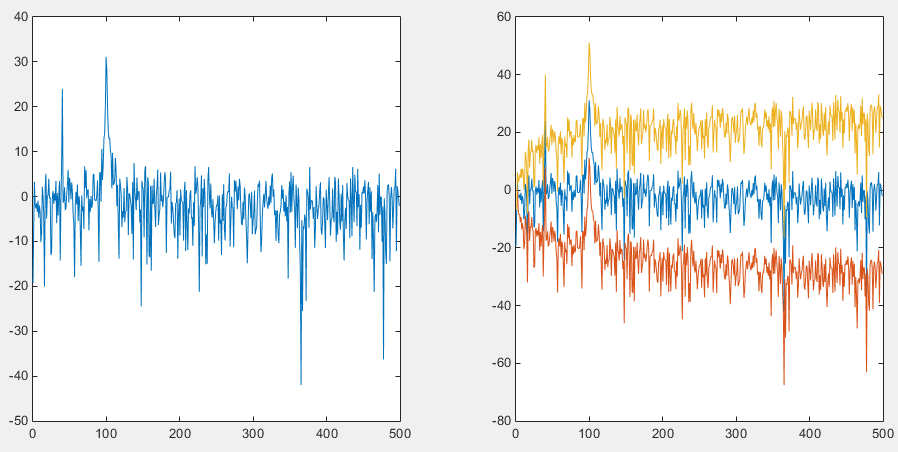4 Welch法
Welch法对Bartlett法进行了两方面的修正，一是选择适当的窗函数

w

(

n

)

w(n)

，并再周期图计算前直接加进去，加窗的优点是无论什么样的窗函数均可使谱估计非负。二是在分段时，可使各段之间有重叠，这样会使方差减小。 Matlab代码示例：
clear;
Fs=1000;
n=0:1/Fs:1;
xn=cos(2*pi*40*n)+3*cos(2*pi*100*n)+randn(size(n));
nfft=1024;
window=boxcar(100); %矩形窗
window1=hamming(100); %海明窗
window2=blackman(100); %blackman窗
noverlap=20; %数据无重叠
range='half'; %频率间隔为[0 Fs/2]，只计算一半的频率
[Pxx,f]=pwelch(xn,window,noverlap,nfft,Fs,range);
[Pxx1,f1]=pwelch(xn,window1,noverlap,nfft,Fs,range);
[Pxx2,f2]=pwelch(xn,window2,noverlap,nfft,Fs,range);
plot_Pxx=10*log10(Pxx);
plot_Pxx1=10*log10(Pxx1);
plot_Pxx2=10*log10(Pxx2);

subplot(1,3,1);
plot(f,plot_Pxx);

subplot(1,3,2);
plot(f1,plot_Pxx1);

subplot(1,3,3);
plot(f2,plot_Pxx2);

结果，从左至右分别为：矩形窗、海明窗、blackman窗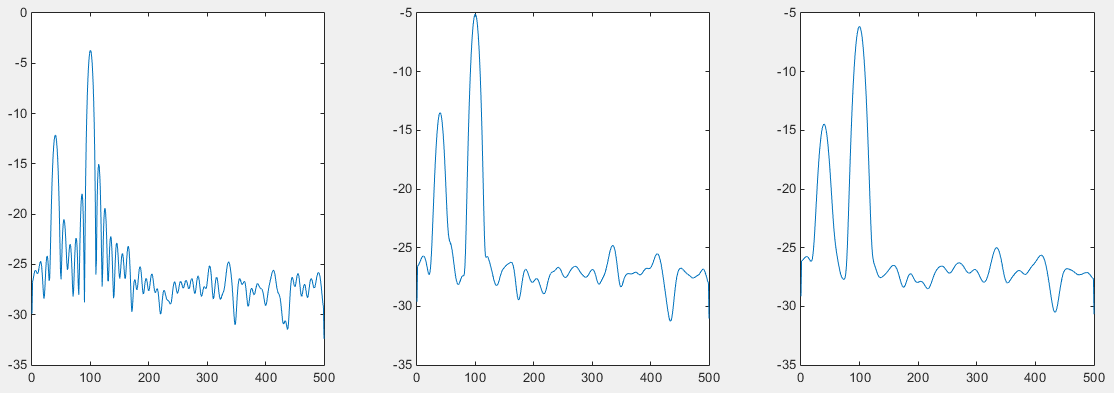附上谋篇论文，分析EEG信号功率谱代码
Matlab 代码：
fs=200;
n=0:1/fs:1;
xn=cos(2*pi*40*n)+cos(2*pi*41*n)+3*cos(2*pi*90*n)+0.1*randn(size(n));
window=boxcar(length(xn));
nfft=512;
[pxx,f]=periodogram(xn,window,nfft,fs);
figure(12);
subplot(121);
plot(f,10*log10(pxx));
xlabel('frequency(hz)');
ylabel('power spectral density(db/hz)');
title('period psd estimate');
orderl=50;
range='half';
magunits='db';
subplot(122);
pburg(xn,orderl,nfft,fs,range);

结果如下：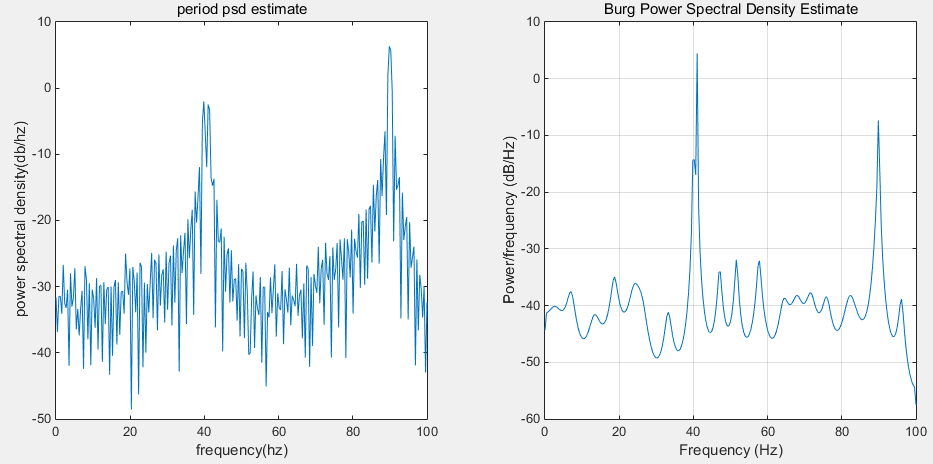致谢


展开全文MATLAB 脑电信号分析
• 经典功率谱估计 Bartlett法 自己编写的程序
• LabVIEW是一种程序开发环境，由美国国家仪器（NI）公司研制开发，类似于C和BASIC开发环境，但是LabVIEW与其他计算机语言的显著区别是：其他计算机语言都是采用基于文本的语言产生代码，而LabVIEW使用的是图形化编辑...
• 直接法得到数据的功率谱，给出的是matlab程序，可以通过修改数据进行应用
• Burg法进行功率谱估计，分别由阶数为15，20和点数分别为1024，512，256等不同的分析 Burg法进行功率谱估计，分别由阶数为15，20和点数分别为1024，512，256等不同的分析
• Python编程实现功率谱估计的平滑改进。首先生成一个随机信号，然后利用周期图法对该有限长度随机信号的功率谱进行计算，为了使得功率谱更为光滑，最后利用Welch法对信号的功率谱进行了平滑改进。
• 原始信号参数设置，波形显示，滤波调节设置，波形幅值显示，波形显示，功率谱计算值显示，经FFT功率谱计算后信号波形显示labview
• 功率谱密度估计方法的MATLAB实现 在应用数学和物理学中谱密度功率谱密度和能量谱密度是一个用于信号的通用概念 它表示每赫兹的功率每赫兹的能量这样的物理量纲在物理学中信号通常是波的形式 例如电磁波随机振动或者...
• 2.随机信号功率谱的估计方法：对于平稳随机信号，其功率谱与其自相关函数为傅里叶变换对。对于本例而言，其均值E[x(n)]为0，自相关函数R(m)与时间起点无关，只与时间间隔m有关因而具有平稳性，可以直接运用维纳-欣钦...
• 通过Matlab，进行22FSK的不同频差的功率谱密度的仿真实现。
• 经典功率谱估计，周期图法功率谱估计（直接法），没用MATLAB自带的函数，自己编写的函数，运行结果和MATLAB自带函数一样
• 3种经典功率谱估计方法的MATLABA代码-功率谱代码.doc 3种MATLAB的经典谱估计方法 希望对大家有用~ 附件所有代码： 直接法： 直接法又称周期图法，它是把随机序列x的N个观测数据视为一能量有限的序列，直接计算x...matlab
• 将进化功率谱密度（EPSD）与Matlab中实现的众所周知的谱图进行了比较。 EPSD 产生更平滑的信号，尤其是在数据点数量较少的情况下。 在下面，我使用  中的示例，其中频谱图适用。 对于其他应用，例如土木工程，...matlab
• 求振动信号功率谱的两种代码，一种周期法，一种AR_pyuler法，支持Excel和text读取
• 功率谱估计，直接法，welch算法求取信号功率谱，包含FFT直接法估计功率谱，求取的结果和matlab的库函数pwelch完全一致，信号默认采用hamming窗，可自行修改。
• 间接法功率谱估计（自相关法），没有MATLAB自带程序，全是自己编写的函数，仿真结果与MATLAB自带函数一样
• 要区分功率谱和能量谱，首先要清楚两种不同类型的信号：功率信号和能量信号。我们从一个具体的物理系统来引出能量信号和功率信号的概念。已知阻值为R的电阻上的电压和电流分别为v(t) 和 i(t)，则此电信号的瞬时功率...
转自：http://longer.spaces.eepw.com.cn/articles/article/item/71979
要区分功率谱和能量谱，首先要清楚两种不同类型的信号：功率信号和能量信号。我们从一个具体的物理系统来引出能量信号和功率信号的概念。已知阻值为R的电阻上的电压和电流分别为v(t) 和 i(t)，则此电信号的瞬时功率为： p(t) = v2(t)/R = i2(t)R。在作定性分析时，为了方便起见，通常假设电阻R为1欧姆而得到归一化 (Normolized) 的功率值。作定量计算时可以通过去归一化，即将实际的电阻值代入即可得到实际的功率值。将上面的概念做一个抽象，对信号 x(t) 定义其瞬时功率为 |f (t)|2，在时间间隔 (-T/2  T/2) 内的能量为:
E=int(|f (t)|2 ,-T/2,T/2)                       (1)
上式表示对|f (t)|2积分，积分限为(-T/2  T/2)。
该间隔内的平均功率为：
p = E/T                                  (2)
当且仅当f(t)在所有时间上的能量不为0且有限时，该信号为能量信号，即(1)式中的 T 趋于无穷大的时候E为有限。典型的能量信号如方波信号、三角波信号等。但是有些信号不满足能量信号的条件，如周期信号和能量无限的随机信号，此时就需要用功率来描述这类信号。当且仅当x(t)在所有时间上的功率不为0且有限时，该信号为功率信号，即 (2) 式中的 T 趋于无穷大的时候 p 为有限。系统中的波形要么具有能量值，要么具有功率值，因为能量有限的信号功率为0，而功率有限的信号能量为无穷大。一般来说，周期信号和随机信号是功率信号，而非周期的确定信号是能量信号。将信号区分为能量信号和功率信号可以简化对各种信号和噪声的数学分析。还有一类信号其功率和能量都是无限的，如 f(t) = t，这类信号很少会用到。
了解了功率信号和能量信号后，功率谱和能量谱就比较好理解了，描述功率信号便使用功率谱，能量信号使用能量谱。所谓的能量谱，也称为能量谱密度，是指用密度的概念表示信号能量在各频率点的分布情况。也即是说，对能量谱在频域上积分就可以得到信号的能量。能量谱是信号幅度谱的模的平方，其量纲是焦/赫。对于功率信号，常用功率谱来描述。所谓的功率谱，也称为功率谱密度，是指用密度的概念表示信号功率在各频率点的分布情况。也就是说，对功率谱在频域上积分就可以得到信号的功率。从理论上来说，功率谱是信号自相关函数的傅里叶变换。因为功率信号不满足傅里叶变换的条件，其频谱通常不存在，维纳-辛钦定理证明了自相关函数和傅里叶变换之间对应关系。在工程实际中，即便是功率信号，由于持续的时间有限，可以直接对信号进行傅里叶变换，然后对得到的幅度谱的模求平方，再除以持续时间来估计信号的功率谱。
展开全文• 本算法允许绘制在运动图像（MI）实验中获得的α和β脑节律的功率谱图。 该算法分为三个阶段： 预处理，α和β振荡的构象以及所述节律的功率谱密度估计。 在采集脑电信号期间，MI阶段由十次试验组成，每个阶段又由...matlab
• 功率谱密度函数 MATLAB代码psdr 概述 作者：Yong-Han Hank Cheng 该软件包允许您生成和比较给定时间序列数据的功率谱密度 (PSD) 图。 快速傅立叶变换 (FFT) 用于获取时间序列数据，分析振荡，然后以 PSD 图的形式...
• 采用fft、小波分析技术，深入分析地震波频谱特性。便于设计院、振动台试验和科研前期分析环节，如问题微信公众号咨询：土木科研咨询团
• ncl对于多维（时间、level、纬度、经度）的数据进行功率谱分析以及基于FFT的带通滤波
• 经验参考设置： 正弦信号测量——VBW/RBW=0.3~1 脉冲信号测量——VBW/RBW=0.1 噪声信号测量——VBW/RBW=9 引用标准： 功率谱密度的测试方法是在EN300328 V1.7.1标准中5.7.3项中明确定义，产品如果出口欧洲，做CE...
• matlab对OFDM（正交频分复用）和FBMC（滤波器组多载波）的功率谱进行仿真，含代码和仿真报告。 对OFDM和FBMC的功率谱进行仿真，加深对OFDM和FBMC的理解，熟悉其系统原理构成。 (The power spectrum density of ......